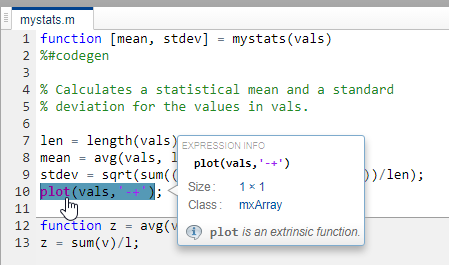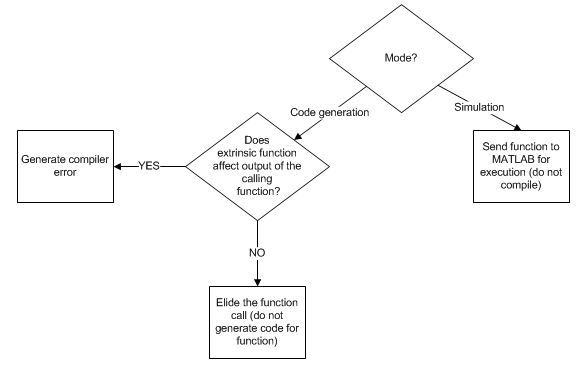## 外部函数### 将 MATLAB 函数声明为外部函数

`coder.extrinsic('function_name_1', ... , 'function_name_n');`

#### 声明外部函数

```function c = pythagoras(a,b,color) %#codegen % Calculates the hypotenuse of a right triangle % and displays the triangle. c = sqrt(a^2 + b^2); create_plot(a, b, color); function create_plot(a, b, color) %Declare patch as extrinsic coder.extrinsic('patch'); x = [0;a;a]; y = [0;0;b]; patch(x, y, color); axis('equal');```

1. 在 MATLAB 提示符下，通过执行以下命令将 `pythagoras` 转换为 MEX 函数：

`codegen -report pythagoras -args {1, 1, [.3 .3 .3]}`

2. 点击代码生成报告的链接，然后在该报告中查看 `create_plot` 的 MATLAB 代码。

该报告突出显示了 `patch``axis` 函数，表明仅在 MATLAB 环境中支持它们。3. 通过执行以下命令运行 MEX 函数：

`pythagoras_mex(3, 4, [1.0 0.0 0.0]);`

MATLAB 将直角三角形的绘图显示为红色补片对象：#### 何时使用 coder.extrinsic 构造

• 调用在仿真过程中不会生成输出，从而不会生成不必要代码的 MATLAB 函数（请参阅在仿真过程中解析外部函数）。

• 使您的代码具有自说明，从而更容易调试。您可以扫描 `coder.extrinsic` 语句的源代码以隔离对 MATLAB 函数的调用，它们可能会创建并传播 `mxArrays`（请参阅使用 mxArray）。

• 节省打字。使用一个 `coder.extrinsic` 语句，就能让每个后续函数调用都是外部调用，只要调用和语句在相同的作用域内（请参阅外部函数声明的作用域）。

• 在整个调用函数作用域内将 MATLAB 函数声明为外部函数（请参阅外部函数声明的作用域）。要缩小作用域，请使用 `feval`（请参阅使用 feval 调用 MATLAB 函数）。

#### 外部函数声明规则

• 在调用函数之前将其声明为外部函数。

• 不要在条件语句中使用外部声明。

#### 外部函数声明的作用域

`coder.extrinsic` 构造具有函数作用域。以如下代码为例：

```function y = foo %#codegen coder.extrinsic('rat','min'); [N D] = rat(pi); y = 0; y = min(N, D); ```

• 在局部函数中将 MATLAB 函数声明为外部函数，如以下示例中所示：

```function y = foo %#codegen coder.extrinsic('rat'); [N D] = rat(pi); y = 0; y = mymin(N, D); function y = mymin(a,b) coder.extrinsic('min'); y = min(a,b); ```

这里，函数 `rat` 每次在主函数 `foo` 内调用时都是外部函数，但函数 `min` 只有在局部函数 `mymin` 内调用时才是外部函数。

• 使用 `feval` 调用 MATLAB 函数，如使用 feval 调用 MATLAB 函数中所述。

### 使用 feval 调用 MATLAB 函数

```function y = foo coder.extrinsic('rat'); [N D] = rat(pi); y = 0; y = feval('min', N, D); ```

### 对非静态方法进行外部声明

• 将对 `foo` 的调用编写为函数调用。不要使用圆点表示法来编写调用。

• 使用语法 `coder.extrinsic('foo')``foo` 声明为外部函数。

```classdef myClass properties prop = 1 end methods function y = foo(obj,x) y = obj.prop + x; end end end ```

```function y = myFunction(x) %#codegen coder.extrinsic('foo'); obj = myClass; y = foo(obj,x); end ```

### 在仿真过程中解析外部函数### 使用 mxArray

• `mxArrays` 存储在变量中

• `mxArrays` 传递给函数，并从函数中返回它们

• 在运行时将 `mxArrays` 转换为已知类型

#### 将 mxArray 转换为已知类型

```function y = foo %#codegen coder.extrinsic('rat'); [N D] = rat(pi); y = min(N, D); ```

`Function output 'y' cannot be of MATLAB type.`

```function y = foo %#codegen coder.extrinsic('rat'); [N D] = rat(pi); y = 0; % Define y as a scalar of type double y = min(N,D);```

### 代码生成中对于外部函数的限制

• 检查调用方或者读取或写入调用方工作区的 MATLAB 函数在代码生成过程中不起作用。此类函数包括：

• MATLAB 调试器无法检查在外部函数中定义的变量。

• 如果您的外部函数在运行时执行以下操作，则生成的代码中的函数可能会产生不可预知的结果：

• 更改文件夹

• 更改 MATLAB 路径

• 删除或添加 MATLAB 文件

• 更改警告状态

• 更改 MATLAB 预设

• 代码生成器不支持使用 `coder.extrinsic` 来调用位于私有文件夹中的函数。

• 代码生成器不支持使用 `coder.extrinsic` 来调用局部函数。